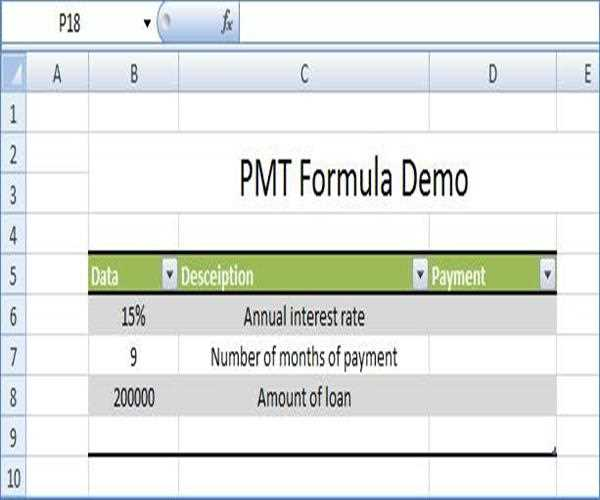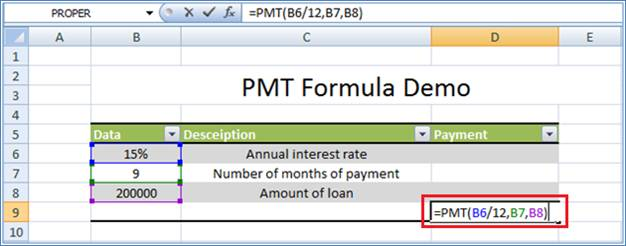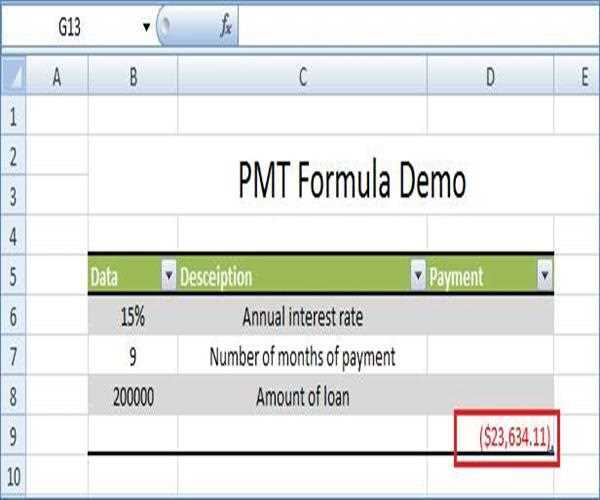# PMT function in Excel

The PMT (Payment) function, one of Excel's financial functions, can be used to calculate the payments for a loan or the future value of an investment. In other words for calculating the payment for a loan based on constant payments and a constant interest rate PMT function used.

##### PMT(rate,nper,pv,fv,type)

Rate     is the interest rate for the loan.

Nper     is the total number of payments for the loan.

Pv     is the present value, or the total amount that a series of future payments is worth now; also known as the principal.

Fv     is the future value, or a cash balance you want to attain after the last payment is made. If fv is omitted, it is assumed to be 0 (zero), that is, the future value of a loan is 0.

Type     is the number 0 (zero) or 1 and indicates when payments are due.

##### Steps are given below

Step 1: First create one excel sheet as belowStep 2:  Now apply PMT formula as below##### Note:

If you make monthly payments on a four-year loan at an annual interest rate of 12 percent, use 12%/12 for rate and 4*12 for nper. If you make annual payments on the same loan, use 12 percent for rate and 4 for nper.

Step 3: Now press enter button and calculate amount of loan payment.Last updated:9/7/2019 12:14:10 AM#### AVADHESH PATEL

Avadhesh Kumar Patel District Project Manager - Aligarh 14 months work experience in Panchayati Raj Department Sector as District Project Manager & 12 months work experience in IT Sector as Software Engineer. :-)